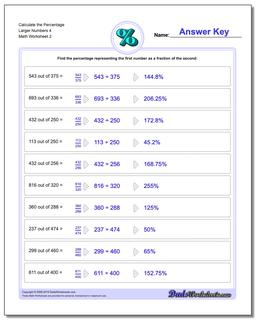# Math Worksheets: Percentages: Percentages: Calculate the Percentage Larger Numbers 4 (Second Worksheet)## Calculate the Percentage Larger Numbers 4 (Second Worksheet)

PropertyValue
DescriptionCalculate the Percentage Larger Numbers 4: Percent worksheets where students are given two numbers and must determine what percentage one number is of the other number. (Second Worksheet)
Resource TypeWorksheet